• 概念最小二乘法（Alternative -Least-Squares）是一种迭代算法。它通过最小化误差的平方和寻找数据的最佳函数匹配。利用最小二乘法可以简便地求得未知的数据，并使得这些求得的数据与实际数据之间误差的平方和为最小...
概念

最小二乘法（Alternative -Least-Squares）是一种迭代算法。它通过最小化误差的平方和寻找数据的最佳函数匹配。利用最小二乘法可以简便地求得未知的数据，并使得这些求得的数据与实际数据之间误差的平方和为最小。最小二乘法可用于曲线拟合。 二乘法曲线拟合：根据给定的n个点,并不要求这条曲线精确地经过这些点，而是曲线y=f(x)的近似曲线y= φ(x)。

原理

给定数n个数据点p(xi,yi)，其中i=1,2,…,n。求近似曲线y= φ(x)。并且使得近似曲线与y=f(x)的偏差最小。近似曲线在点p处的偏差δi= φ(xi)-yi，i=1,2,…,n。

常见的拟合方法有：

使偏差绝对值之和最小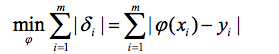使偏差绝对值最大的最小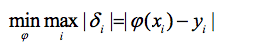使偏差平方和最小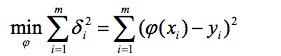按偏差平方和最小的原则选取拟合曲线，并且采取二项式方程为拟合曲线的方法，称为最小二乘法

推导过程

设拟合多项式为：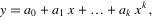各点到这条曲线的距离之和，即偏差平方和如下：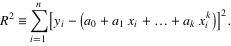为了求得符合条件的a值，对等式右边求ai偏导数，因而我们得到了：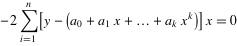将等式左边进行一下化简，然后应该可以得到下面的等式：
把这些等式表示成矩阵的形式，就可以得到下面的矩阵：
将这个范德蒙得矩阵化简后可得到:
也就是说X*A=Y，那么A = (X’*X)-1*X’*Y，便得到了系数矩阵A，同时，我们也就得到了拟合曲线。
实现
展开全文算法 迭代 函数
• 由于Z-I关系的非线性，线性回归模型中常规最小二乘法的一些基本假设在拟合过程中无法满足。研究表明使用不同的计算方法，同样的数据可能得到差异明显的A、b值。本文从常规最小二乘法基本假设入手，通过2006年大连...
• 普通最小二乘法（ordinary least squares, OLS）是线性回归预测问题中一个很重要的概念，在 Introductory Econometrics A Modern Approach (Fourth Edition) 第2章 简单回归模型 中，花了很详细的篇幅对此作出介绍。
• 对应的是菜单中“最小二乘法”的那个。过程辨识，就是根据一个模型的输入数据及对应的输出数据(采样值，非连续)来预测下一个输入采样点对应的输出会是多少。最后辨识出来的结果是形如下面的表达式：y(k)+a1*y(k-1)+...
• 最小二乘法：使平方误差最小。相比于梯度下降法，最小二乘法有解析形式的解，比较直观。 本文主要讨论线性回归模型中最小二乘法求解...附：求解过程中用到的一些代数知识（tr表示矩阵的迹，即一个方阵对角线元素之和）
最小二乘法：使平方误差最小。有两种方法：线性和非线性。
线性最小二乘的解存在解析的形式，是closed-form，比较直观，就是等下要推导的……
非线性最小二乘的解没有closed-form，是要通过迭代来求得，比如梯度下降法……

本文主要讨论线性回归模型中最小二乘法线性解法的推导。
参考：Andrew Ng《机器学习》第二集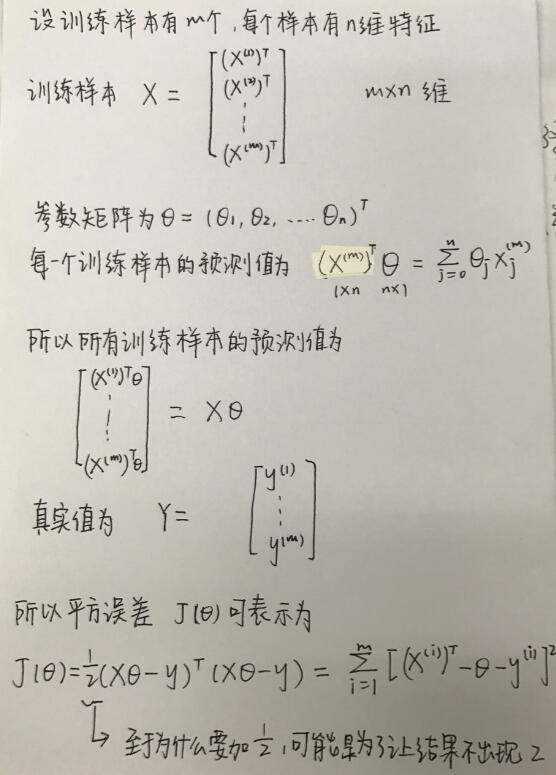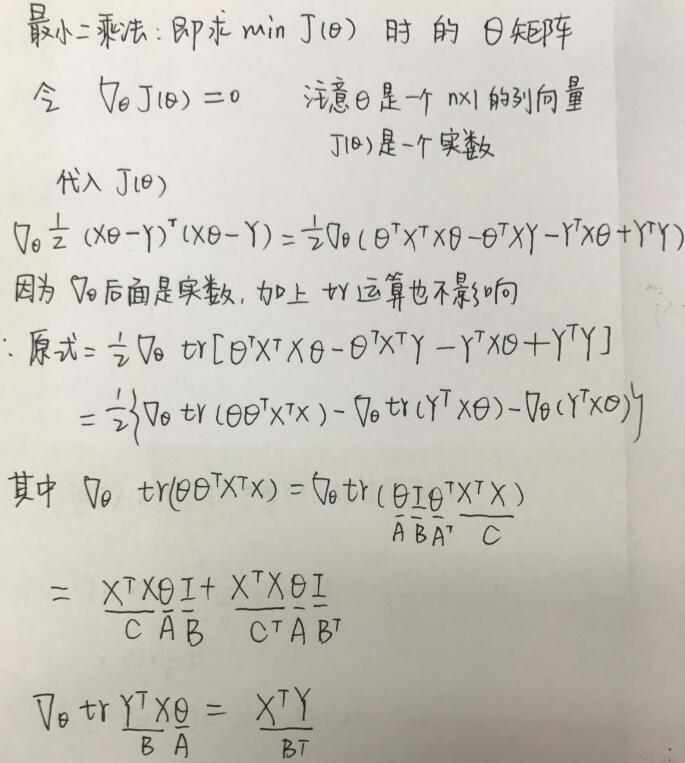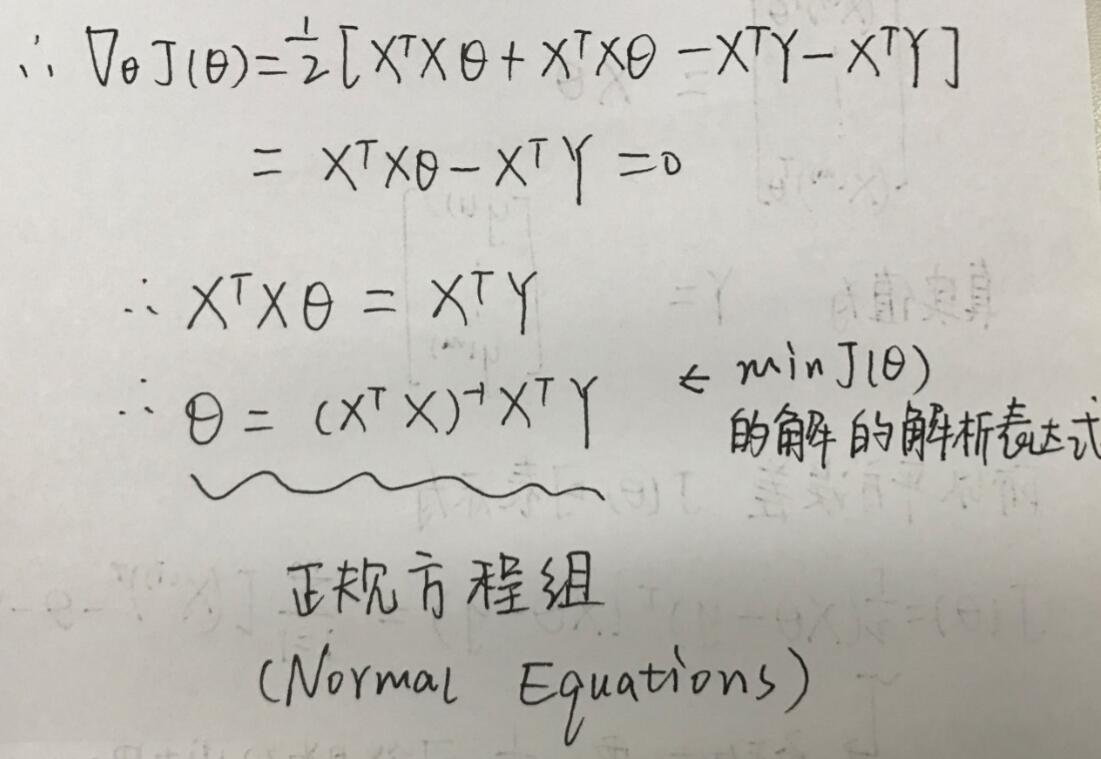附：求解过程中用到的一些代数知识（tr表示矩阵的迹，即一个方阵对角线元素之和）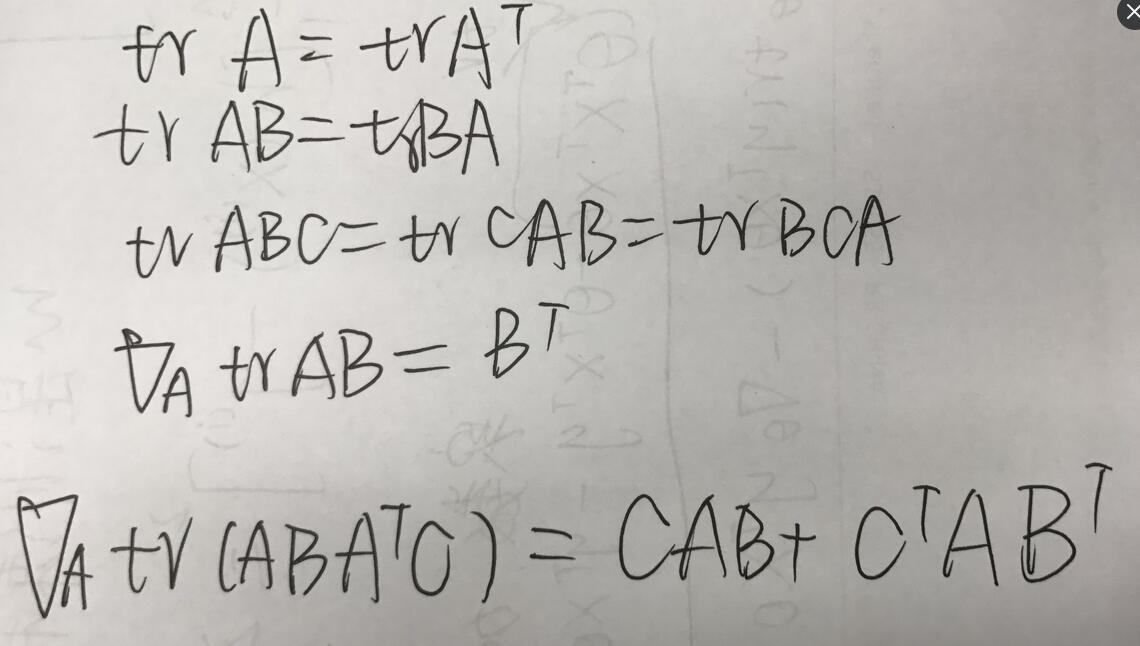展开全文机器学习
• 最小二乘法是由勒让德在19世纪发现的，原理的一般形式很简单，当然发现的过程是非常艰难的。形式如下式： 目标函数 = Σ（观测值-理论值) 2^22 观测值就是我们的多组样本，理论值就是我们的假设拟合函数。目标函数也...
最小二乘法是用来做函数拟合或者求函数极值的方法。在机器学习，尤其是回归模型中，经常可以看到最小二乘法的身影，这里就对我对最小二乘法的认知做一个小结。
1.最小二乘法的原理与要解决的问题
最小二乘法是由勒让德在19世纪发现的，原理的一般形式很简单，当然发现的过程是非常艰难的。形式如下式：
目标函数 = Σ（观测值-理论值) $^2$
观测值就是我们的多组样本，理论值就是我们的假设拟合函数。目标函数也就是在机器学习中常说的损失函数，我们的目标是得到使目标函数最小化时候的拟合函数的模型。举一个最简单的线性回归的简单例子，比如我们有m个只有一个特征的样本：
$(x^{(1)},y^{(1)}),(x^{(2)},y^{(2)}),...(x^{(m)},y^{(m)})$
样本采用下面的拟合函数：
$h_\theta(x)=\theta_0+\theta_1x$
这样我们的样本有一个特征x，对应的拟合函数有两个参数$\theta_0$和$\theta_1$需要求出。
我们的目标函数为：
$J(\theta_0,\theta_1)=\sum_{i=1}{m}( y^{(i)}-\theta_0-\theta_1x^{(i)})2$
用最小二乘法做什么呢，使$J(\theta_0,\theta_1)$最小，求出使$J(\theta_0,\theta_1)$最小时的$\theta_0$和$\theta_1$，这样拟合函数就得出了。
那么，最小二乘法怎么才能使$J(\theta_0,\theta_1)$最小呢？
2.最小二乘法的代数法解法
上面提到要使$J(\theta_0,\theta_1)$最小，方法就是对$\theta_0$和$\theta_1$分别来求偏导数，令偏导数为0，得到一个关于$\theta_0$和$\theta_1$的二元方程组。求解这个二元方程组，就可以得到$\theta_0$和$\theta_1$的值。下面我们具体看看过程。
$J($$\theta_0$$,$$\theta_1$$)对$$\theta_0$求导，得到如下方程：
$\sum\limits_{i=1}{m}(y^{(i)} - \theta_0 - \theta_1 x^{(i)}) = 0$
$J(\theta_0, \theta_1)$对$\theta_1$求导，得到如下方程：
$\sum\limits_{i=1}{m}( y^{(i)} - \theta_0 - \theta_1 x^{(i)})x^{(i)} = 0$
①和②组成一个二元一次方程组，容易求出$\theta_0$和$\theta_1$的值：
$\theta_0 = \sum\limits_{i=1}{m}\big( x^{(i)})2\sum\limits_{i=1}{m}y^{(i)} - \sum\limits_{i=1}{m}x^{(i)}\sum\limits_{i=1}{m}x^{(i)}y^{(i)} \Bigg/ n\sum\limits_{i=1}{m}\big(x^{(i)})^2 - \big(\sum\limits_{i=1}{m}x^{(i)})^2$
$\theta_1 = n\sum\limits_{i=1}{m} x^{(i)}y^{(i)} - \sum\limits_{i=1}{m}x^{(i)}\sum\limits_{i=1}{m}y^{(i)} \Bigg/ n\sum\limits_{i=1}{m}\big(x^{(i)})^2 - \big(\sum\limits_{i=1}{m}x^{(i)})^2$
这个方法很容易推广到多个样本特征的线性拟合。
拟合函数表示为 $h_\theta(x_1, x_2, ...x_n) = \theta_0 + \theta_{1}x_1 + ... + \theta_{n}x_{n}$, 其中$\theta_i$(i = 0,1,2… n)为模型参数，$x_i$(i = 0,1,2… n)为每个样本的n个特征值。这个表示可以简化，我们增加一个特征$x_0=1$，这样拟合函数表示为：
$h_\theta(x_0, x_1, ...x_n) = \sum\limits_{i=0}^{n}\theta_{i}x_{i}$
损失函数表示为：
$J(\theta_0, \theta_1..., \theta_n) = \sum\limits_{j=1}^{m}(h_\theta(x_0^{(j)}), x_1^{(j)}, ...x_n^{(j)})) - y^{(j)}))2 = \sum\limits_{j=1}^{m}(\sum\limits_{i=0}^{n}\theta_{i}x_{i}^{(j)}- y^{(j)})2$
利用损失函数分别对$\theta_i$(i=0,1,…n)求导,并令导数为0可得：
$\sum\limits_{j=0}^{m}(\sum\limits_{i=0}^{n}\theta_{i}x_{i}^{(j)} - y_j)x_i^{j}=0$ (i=0,1,…n)
这样我们得到一个N+1元一次方程组，这个方程组有N+1个方程，求解这个方程，就可以得到所有的N+1个未知的θ。
这个方法很容易推广到多个样本特征的非线性拟合。原理和上面的一样，都是用损失函数对各个参数求导取0，然后求解方程组得到参数值。这里就不累述了。
3.最小二乘法的矩阵法解法
矩阵法比代数法要简洁，且矩阵运算可以取代循环，所以现在很多书和机器学习库都是用的矩阵法来做最小二乘法。
这里用上面的多元线性回归例子来描述矩阵法解法。
假设函数$h_\theta(x_1, x_2, ...x_n) = \theta_0 + \theta_{1}x_1 + ... + \theta_{n}x_{n}$的矩阵表达方式为：
$h_\mathbf{\theta}(\mathbf{x}) = \mathbf{X\theta}$
其中， 假设函数$h_\mathbf{\theta}(\mathbf{X})$为mx1的向量,θ为nx1的向量，里面有n个代数法的模型参数。X为mxn维的矩阵。m代表样本的个数，n代表样本的特征数。
损失函数定义为$J(\mathbf\theta) = \frac{1}{2}(\mathbf{X\theta} - \mathbf{Y})^T(\mathbf{X\theta} - \mathbf{Y})$
其中Y是样本的输出向量，维度为mx1. 1/2在这主要是为了求导后系数为1，方便计算。
根据最小二乘法的原理，我们要对这个损失函数对θ向量求导取0。结果如下式：
$\frac{\partial}{\partial\mathbf\theta}J(\mathbf\theta) = \mathbf{X}^T(\mathbf{X\theta} - \mathbf{Y}) = 0$
这里面用到了矩阵求导链式法则，和两个矩阵求导的公式。
公式1：$\frac{\partial}{\partial\mathbf{X}}(\mathbf{XX^T}) =2\mathbf{X}$
公式2：$\frac{\partial}{\partial\mathbf\theta}(\mathbf{X\theta}) =\mathbf{X^T}$
对上述求导等式整理后可得：
$\mathbf{x^{T} X\theta} = \mathbf{x^{T}Y}$
两边同时左乘$(\mathbf{x^{T} X}){-1}$可得：
$\mathbf{\theta} = (\mathbf{X^{T}X})^{-1}\mathbf{X^{T}Y}$
这样我们就一下子求出了θ向量表达式的公式，免去了代数法一个个去求导的麻烦。只要给了数据,我们就可以用$\mathbf{\theta} = (\mathbf{X^{T}X})^{ -1}\mathbf{X^{T}Y}$算出θ。
4.最小二乘法的局限性和适用场景
从上面可以看出，最小二乘法适用简洁高效，比梯度下降这样的迭代法似乎方便很多。但是这里我们就聊聊最小二乘法的局限性。
首先，最小二乘法需要计算$\mathbf{X^{T}X}$的逆矩阵，有可能它的逆矩阵不存在，这样就没有办法直接用最小二乘法了，此时梯度下降法仍然可以使用。当然，我们可以通过对样本数据进行整理，去掉冗余特征。让$\mathbf{X^{T}X}$的行列式不为0，然后继续使用最小二乘法。
第二，当样本特征n非常的大的时候，计算$\mathbf{X^{T}X}$的逆矩阵是一个非常耗时的工作（nxn的矩阵求逆），甚至不可行。此时以梯度下降为代表的迭代法仍然可以使用。那这个n到底多大就不适合最小二乘法呢？如果你没有很多的分布式大数据计算资源，建议超过10000个特征就用迭代法吧。或者通过主成分分析降低特征的维度后再用最小二乘法。
第三，如果拟合函数不是线性的，这时无法使用最小二乘法，需要通过一些技巧转化为线性才能使用，此时梯度下降仍然可以用。
第四，讲一些特殊情况。当样本量m很少，小于特征数n的时候，这时拟合方程是欠定的，常用的优化方法都无法去拟合数据。当样本量m等于特征数n的时候，用方程组求解就可以了。当m大于n时，拟合方程是超定的，也就是我们常用与最小二乘法的场景了。


展开全文• <1>假设拟合直线为： <2>平均损失函数： 注：（xi,yi）是样本点。 ...要使得拟合直线拟合很充分的话，平均损失函数应该取得最小值。可以分别对W0, W1求偏导，然后...求解过程如下手工计算步骤： ...


<1>假设拟合直线为：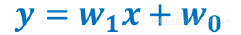<2>平均损失函数：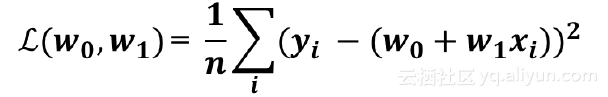注：（xi,yi）是样本点。
<3>要使得拟合直线拟合很充分的话，平均损失函数应该取得最小值。可以分别对W0, W1 求偏导，然后将两个偏导函数等于0，求得W0, W1 。求解过程如下手工计算步骤：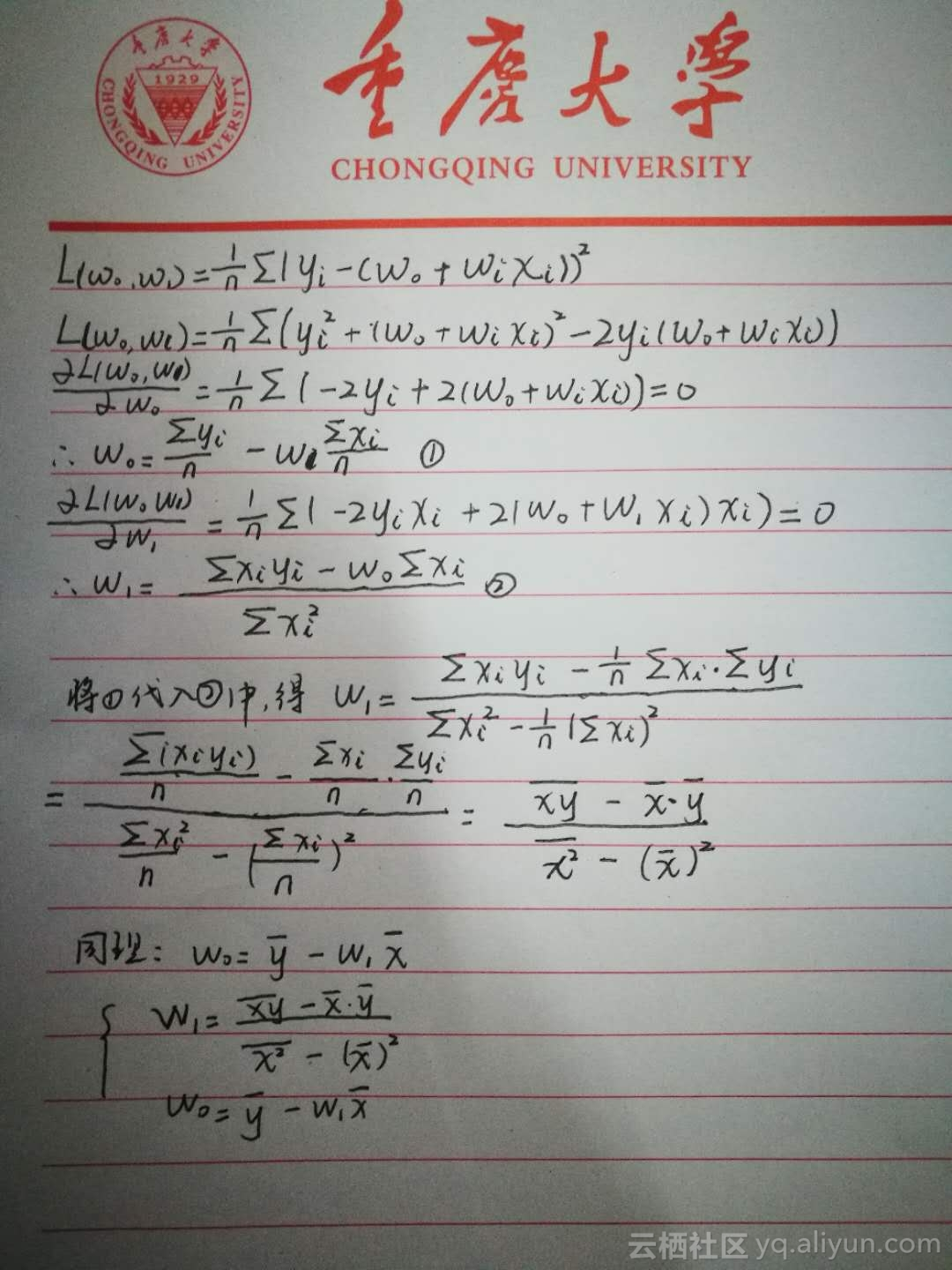展开全文• **1.2 最小二乘法：**基于L2范数（均方误差）最小化进行模型求解的方法。 2.计算过程 假设输入特征的值只有一个： 在线性回归中，最小二乘法就是找到一条直线，使所有样本到这条直线的距离之和最小。 求解!w和b，...机器学习 大数据 人工智能
• 有四种方法可以处理违背回归假设的问题 1，删除观测点 2，变量变换 ...若离群点或强影响点仍然存在，重复以上过程 如果是因为数据记录错误，或没有遵守规程，或是受式对象误解指导说明这种数据本身是错误的...
• 最小二乘法小结 ...　最小二乘法是由勒让德在19世纪发现的，原理的一般形式很简单，当然发现的过程是非常艰难的。形式如下式： 目标函数=∑（观测值−理论值）2目标函数=∑（观测值−理论值）2 　...机器学习
• 1.最小二乘法的原理与要解决的问题　最小二乘法是由勒让德在19世纪发现的，原理的一般形式很简单，当然发现的过程是非常艰难的。形式如下式：　目标函数 = Σ（观测值-理论值）2　观测值就是我们的多组样本，理论.....
• 最小二乘法是由勒让德在19世纪发现的，原理的一般形式很简单，发现的过程是非常艰难的。形式如下式： 观测值就是我们的多组样本，理论值就是我们的假设拟合函数。 目标函数也就是在机器学习中常说的损失函数. ...
• 其基本思想是基于对数据先进行一次滤波处理，后利用普通最小二乘法对滤波后的数据进行辨识，进而获得无偏一致估计。但是当过程的输出信噪比比较大或模型参数较多时，这种数据白色化处理的可靠性就会下降，辨识结果...
• 最小二乘法（Least squares）   最小二乘法是用来做函数...  最小二乘法是由勒让德在 19 世纪发现的，原理的一般形式很简单，当然发现的过程是非常艰难的。形式如下式： 目标函数=∑(观测值−理论值)2目标函数...
• 接上篇文章：机器学习第一期（上）：数学矩阵前置知识、机器学习前置知识、机器学习基础、机器学习类型，分类回归、机器学习过程、损失函数、过拟合与欠拟合、正则化项、训练误差与测试误差、模型选择、交叉验证、...机器学习 机器学习算法 线性回归 逻辑回归 决策树
• 在统计学中，普通最小二乘法（Ordinary Least Squares，OLS）是一种用于在线性回归模型中估计未知参数的线性...一元线性回归求解过程 我们先以一元线性模型为例来说明。 假设有一组数据X={(x1,y1,⋯ ,(xm,ym)}X=\{(...
• 当退化模型可以写成未知参数的线性结合的形式时，去找到未知参数的回归过程就被称为是线性最小二乘法；当退化模型的参数是模型参数的非线性结构时，这回归过程被称为非线性最小二乘法。换句话说，与模型参数或系数......